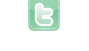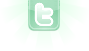## How to fix shaking objects

I've been trying to make an object move left and right when it hits the world border but when it gets the the edge it starts shaking. If someone could tell me how to fix this, that would be helpful.
```public void act()
{
moveLeftAndRight(5);
}
private void moveLeftAndRight(int direction)
{
if((direction < 0 && getX()== 0) || (direction > 0 && getX() == 999)){
direction = -direction;
}
move(direction);
}      ```
Armetor wrote...
I've been trying to make an object move left and right when it hits the world border but when it gets the the edge it starts shaking. If someone could tell me how to fix this, that would be helpful. << Code Omitted >>
Please provide the entire code of the class.
There wasn't much else in the actor code
```/**
* Write a description of class target here.
*
* @version (a version number or a date)
*/
public class target extends Actor
{
/**
* Act - do whatever the target wants to do. This method is called whenever
* the 'Act' or 'Run' button gets pressed in the environment.
*/
public void act()
{
moveLeftAndRight(5);
}
private void moveLeftAndRight(int direction)
{
if((direction < 0 && getX()== 0) || (direction > 0 && getX() == 999)){
direction = -direction;
}
move(direction);
}
}
```
Armetor wrote...
There wasn't much else in the actor code << Code Omitted >>
The problem is that every time the moveLeftAndRight method is called, direction always comes in at positive 5. So, even if it is changed to negative 5 in the method, it is positive 5 on the next call to the method; hence, causing the shaking. The direction variable needs to be retained, so that its last set value is known:
```private int speed = 5;

public void act()
{
moveLeftAndRight();
}

private void moveLeftAndRight()
{
if ((speed < 0 && getX() == 0) || (speed > 0 && getX() == 999))
{
speed = -speed;
}
move(speed);
}```
I renamed the variable to speed to better describe what the value represents.
Ohhhh ok that makes sense. Thank you so much!